StatLect

Joint characteristic function

In the lecture entitled Characteristic function we have introduced the concept of characteristic function (cf) of a random variable. This lecture is about the joint cf, a concept which is analogous, but applies to random vectors.

Definition Letbe arandom vector. The joint characteristic function ofis a functiondefined bywhereis the imaginary unit.

Observe thatexists for anybecauseand the expected values appearing in the last line are well-defined, because both the sine and the cosine are bounded (they take values in the interval).Deriving cross-moments

Like the joint moment generating function of a random vector, the joint cf can be used to derive the cross-moments of, as stated below.

Proposition Letbe a random vector andits joint characteristic function. Let. Define a cross-moment of orderas follows:whereand. If all cross-moments of orderexist and are finite, then all the-th order partial derivatives ofexist and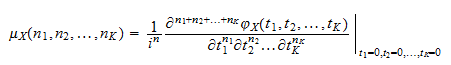where the partial derivative on the right-hand side of the equation is evaluated at the point,, ...,.

Proof

When we need to derive a cross-moment of a random vector, the practical usefulness of this proposition is somewhat limited, because it is seldom known, a priori, whether cross-moments of a given order exist or not. The following proposition, instead, does not require such a priori knowledge.

Proposition Letbe a random vector andits joint cf. If all the-th order partial derivatives ofexist, then

1. ifis even, for anyall-th cross-moments ofexist and are finite;

2. ifis odd, for anyall-th cross-moments ofexist and are finite.

In both cases, we have that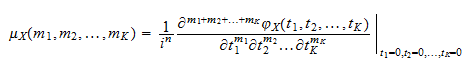where the partial derivatives on the right-hand sides of the equations above are evaluated at the point,, ...,.

Proof

Again, see Ushakov (1999).

Characterizing joint distributions

The joint cf can also be used to check whether two random vectors have the same distribution.

Proposition Letandbe tworandom vectors. Denote byandtheir joint distribution functions and byandtheir joint cfs. Then,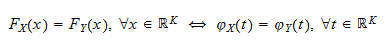Proof

Stated differently, two random vectors have the same distribution if and only if they have the same joint cf. This result is frequently used in applications, because demonstrating equality of two joint cfs is often much easier than demonstrating equality of two joint distribution functions.

More details

The following sections contain more detail about the joint characteristic function.

Joint cf of a linear transformation

Letbe arandom vector with characteristic function. Definewhereis aconstant vector andis aconstant matrix. Then, the joint cf ofisProof

This is proved as follows:Joint cf of a random vector with independent entries

Letbe arandom vector. Let its entries, ...,bemutually independent random variables. Denote the cf of the-th entry ofby.

Then, the joint cf ofisProof

This is demonstrated as follows: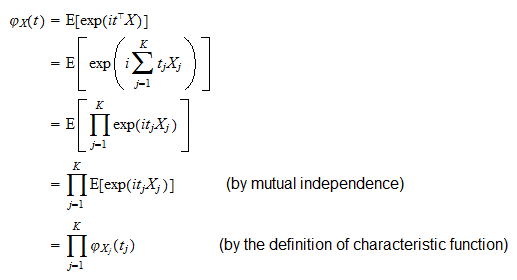Joint cf of a sum of mutually independent random vectors

Let, ...,bemutually independent random vectors. Letbe their sum:Then, the joint cf ofis the product of the joint cfs of, ...,:Proof

Similar to the previous proof: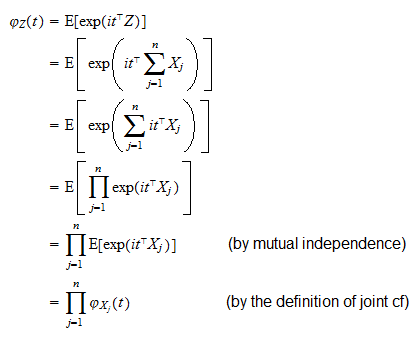Solved exercises

Some solved exercises on joint characteristic functions can be found below.

Exercise 1

Letandbe two independent standard normal random variables. Letbe arandom vector whose components are defined as follows:Derive the joint characteristic function of.

Hint: use the fact thatandare two independent Chi-square random variables having characteristic functionSolution

By using the definition of characteristic function, we get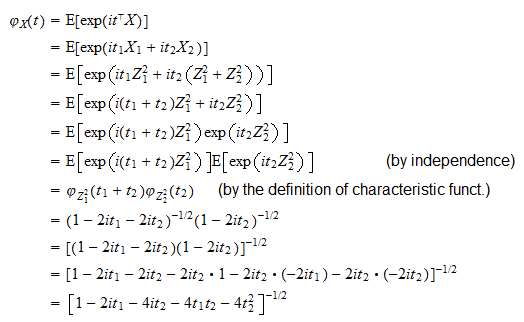Exercise 2

Use the joint characteristic function found in the previous exercise to derive the expected value and the covariance matrix of.

Solution

We need to compute the partial derivatives of the joint characteristic function: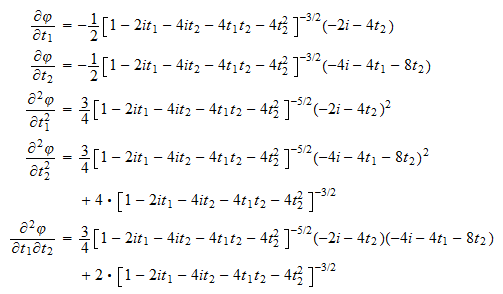All partial derivatives up to the second order exist and are well defined. As a consequence, all cross-moments up to the second order exist and are finite and they can be computed from the above partial derivatives: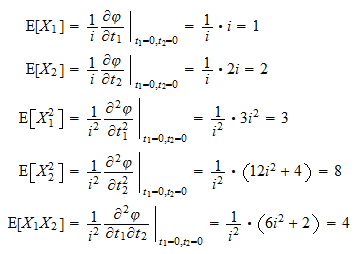The covariances are derived as follows:So, summing up, we get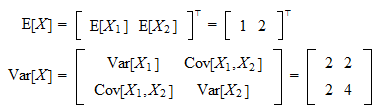Exercise 3

Read and try to understand how the joint characteristic function of the multinomial distribution is derived in the lecture entitled Multinomial distribution.

References

Ushakov, N. G. (1999) Selected topics in characteristic functions, VSP.

The book

Most of the learning materials found on this website are now available in a traditional textbook format.

Glossary entries
Share